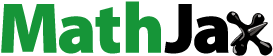# Beautiful Mathematics in All Browsers

I recently discovered the wonderful MathJax. MathJax is a JavaScript library that displays mathematical formulas in web pages. It's very easy to set up and it renders beautiful mathematics.For example:

Suppose $$U$$ is an open subset of the complex plane $$\mathbb{C}$$, $$f : U \mathbb{C}$$ a holomorphic function, and the closed disk $$D = \{z : |z z_0| r\}$$ is completely contained in $$U$$. Let $$\gamma$$ be the circle forming the boundary of $$D$$. Then for every a in the interior of $$D$$:

$$f(a) = \frac{1}{2 \pi i} \oint_{\gamma}{ \frac{f(z)}{z-a} dz}$$

where the contour integral is taken counter-clockwise.

That is, of course, Cauchy's integral formula.

Written on August 3, 2012